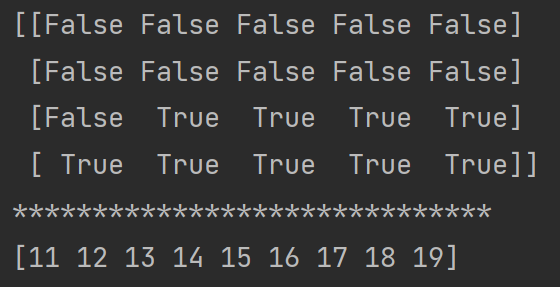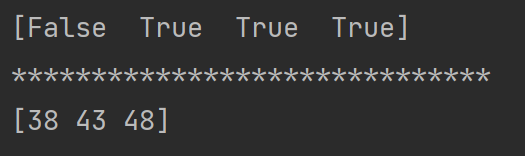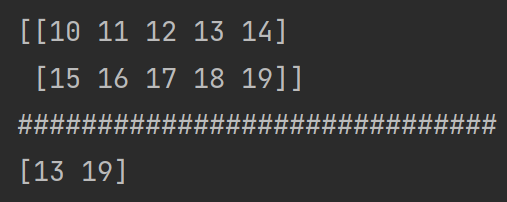WHCSRL 技术网

# numpy学习笔记03—对数组使用索引查询_weixin

``import  numpy as np a = np.array([[0,1,2,3,4],              [5,6,7,8,9],              [10,11,12,13,14],              [15,16,17,18,19]])print(a)print(a[0,0])#0行0列print(a)#2行所有列print(a[0:2,2:4])#取0、1行，2、3列``

``import  numpy as np a = np.array([[0,1,2,3,4],              [5,6,7,8,9],              [10,11,12,13,14],              [15,16,17,18,19]])# 设定筛选规则b = a>10 print(b)print('*'*30)print(a[b])````import  numpy as np a = np.array([[0,1,2,3,4],              [5,6,7,8,9],              [10,11,12,13,14],              [15,16,17,18,19]])# 设定筛选规则b = a[:,3]>5#打印规则，结果为布尔类型print(b)print('*'*30)# 符合筛选规则的数据加30，注意先定位数据（a[:,3]）再执行筛选规则（a[:,3][b]）a[:,3][b]+=30print(a[:,3][b])````import  numpy as np a = np.array([[0,1,2,3,4],              [5,6,7,8,9],              [10,11,12,13,14],              [15,16,17,18,19]])# 打印第二、三行print(a[[2,3]])print('#'*30)# 打印第[2,3]、[3,4]个元素print(a[[2,3],[3,4]])``